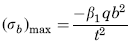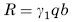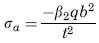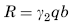Related Resources: calculators

### Uniform over 1/3 of Plate from Fixed Edge Equation and Calculator

Flat Rectangular Plate, Three Edges Fixed, One Edge (a) Free Loading Uniform over 1/3 of Plate from Fixed Edge Equation and Calculator.

Per. Roarks Formulas for Stress and Strain for flat plates with straight boundaries and constant thickness

Flat Rectangular plate, Three Edges Fixed, One Edge Loading Uniform over 1/3 of Plate from Fixed Edge.

 Rectangular plate; Three Edges Fixed One Edge FreeLoading Uniform over 1/3 of Plate from Fixed EdgeAt x = 0, z = 0
Stress and Reaction ForceReaction ForceStress and Reaction Force at x = ± a/2, z = 0.2bReaction ForceWhere used:
E = Modulus of Elasticity (lbs/in2)
q = Total load or force to Plate (lbs/in2)
v = Poisson’s ratio (assumed to be 0.3)
t = plate thickness, (in)
a = plate length, (in)
b = plate width, (in)
σb = stress, (lbs/in2)
R = Reaction Force (lbs/in)
β1,2 = Constant From Table A
γ1,2 = Constant From Table A

Table A

 a/b 0.25 0.5 0.75 1 1.5 2 3 β1 0.02 0.068 0.11 0.148 0.202 0.24 0.29 β2 0.026 0.063 0.084 0.079 0.068 0.057 0.04 γ1 0.115 0.21 0.257 0.291 0.316 0.327 0.335 γ2 0.111 0.17 0.194 0.185 0.174 0.17 0.18

Reference:

Roarks Formulas for Stress and Strain, 7th Edition